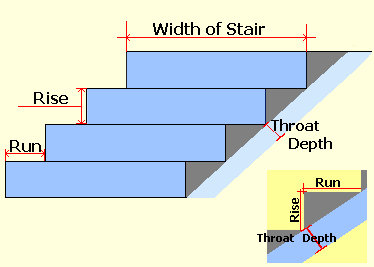Spike's Calculators

# Concrete Steps (Stairs)Calculate the amount of concrete needed for stairs on a slope between two elevations. The volume is calculated from the stair riser height, the run length, the stairs width, and the throat depth of the stairs. The number of risers equals the number of steps.

This calculator uses inches for measurements.### Concrete Steps - Inches

Number of Steps #
Rise in
Run in
Throat Depth of Stairs in
Width of Stairs in
Total Rise in
Total Run in
Stair Slope °
Cubic Feet ft³
Cubic Yards yd³
Cubic Meters

#### Calculation

1. enter the number of steps
2. the riser height of the step(s) in inches
3. the run of the step(s) in inches
4. the thickness (depth) of the throat in inches
5. the width of the stairs in inches

#### Results

1. the total rise of the step(s) in inches
2. total run of the step(s) in inches
3. the slope of the stairs in degrees
4. the amount of concrete needed in cubic feet
5. in cubic yards
6. in cubic metres

##### Conversions
```one cubic inch (in³) = 0.000578703703703704 cubic feet (ft³)
one cubic inch (in³) = 0.0000214334705075445 cubic yards (yd³)
one cubic inch (in³) = 0.000016387064 cubic metres (m³)```

```To convert cubic feet to cubic yards multiply cubic feet * 0.037037037037037
To convert cubic feet to cubic metres multiply cubic feet * 0.028316846592
To convert cubic yards to cubic metres multiply cubic yards * 0.764554857984```

### When Ordering Concrete

This concrete calculator will help you in estimating the amount of concrete needed for your project. The amount given as needed, does not include any waste. It is recommended, depending on the job you are doing, to add anywhere from an extra 4% to 10% to your concrete order to make sure you have enough concrete to finish the job. Ask when ordering the concrete. They will be able to give you a good idea of what is needed.